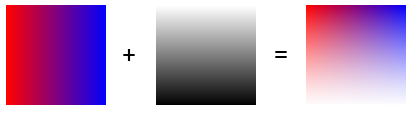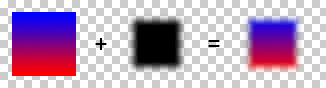A Flash Developer Resource Site

1. ##Apply alpha mask on BitmapData.

Hello,

I try to do this with BitmapData :I have an image 1 ( the red-blue gradient ) , I have an image 2 ( transparent-black gradient ) , and I want to "mix" the 2 images.

So the image 2 is the alpha mask of image 1. Like in Photoshop.

Is it possible to create this with BitmapData ?

I don't know if the image 2 must be a ( white to black gradient ) or a ( transparent to black gradient ) to perform this operation.

Actually I can't find solution. Any idea ?

Code:
```import flash.geom.Matrix;
import flash.display.BitmapData;

// IMAGE 1

var mat = new Matrix();

var d = this.getNextHighestDepth();
var mc1 = this.createEmptyMovieClip("mc_" + d, d);
var colors = [0xFF0000, 0x0000FF];
var alphas = [100, 100];
var ratios = [0, 0xFF];
mc1.lineTo(0, 100);
mc1.lineTo(100, 100);
mc1.lineTo(100, 0);
mc1.lineTo(0, 0);

// IMAGE 2

var mat = new Matrix();

var d = this.getNextHighestDepth();
var mc2 = this.createEmptyMovieClip("mc_" + d, d);
mc2._x = 150
var colors:Array = [0x0, 0x0];
var alphas:Array = [0, 100];
var ratios:Array = [0, 0xFF];
mc2.lineTo(0, 100);
mc2.lineTo(100, 100);
mc2.lineTo(100, 0);
mc2.lineTo(0, 0);

// IMAGE 3

var d = this.getNextHighestDepth();
var mc3 = this.createEmptyMovieClip("mc_" + d, d);
mc3._x = 300
bmp = new BitmapData( mc2._width , mc2._height , true , 0 );
bmp.draw( mc2 )
// mix operation here...
mc3.attachBitmap(bmp,1)```Reply With Quote

Code:
```import flash.geom.Matrix;

// IMAGE 1

var mat = new Matrix();

var d = this.getNextHighestDepth();
var mc1 = this.createEmptyMovieClip("mc_" + d, d);
var colors = [0xFF0000, 0x0000FF];
var alphas = [100, 100];
var ratios = [0, 0xFF];
mc1.lineTo(0, 100);
mc1.lineTo(100, 100);
mc1.lineTo(100, 0);
mc1.lineTo(0, 0);

// IMAGE 2

var mat = new Matrix();

var d = this.getNextHighestDepth();
var mc2 = this.createEmptyMovieClip("mc_" + d, d);
var colors:Array = [0x0, 0x0];
var alphas:Array = [100, 0];
var ratios:Array = [0, 0xFF];
mc2.lineTo(0, 100);
mc2.lineTo(100, 100);
mc2.lineTo(100, 0);
mc2.lineTo(0, 0);

mc1.cacheAsBitmap = mc2.cacheAsBitmap = true;Reply With Quote

3. Yes it works like this, thanksBut I try to do it only by code, not with the use of the timeline.

Is it possible to do it by code ? With BitmapData.applyFilter or other ?Reply With Quote

4. the above example does it in code.Reply With Quote

5. You can also copy channels across bitmap data objects, this example basically gives you the same effect but by using bitmap data objects instead of a mask...

Code:
```import flash.geom.Matrix;
import flash.display.BitmapData;
import flash.geom.Rectangle;
import flash.geom.Point;

// IMAGE 1

var mat = new Matrix();

var d = this.getNextHighestDepth();
var mc1 = this.createEmptyMovieClip("mc_" + d, d);
var colors = [0xFF0000, 0x0000FF];
var alphas = [100, 100];
var ratios = [0, 0xFF];
mc1.lineTo(0, 100);
mc1.lineTo(100, 100);
mc1.lineTo(100, 0);
mc1.lineTo(0, 0);

// IMAGE 2

var mat = new Matrix();

var d = this.getNextHighestDepth();
var mc2 = this.createEmptyMovieClip("mc_" + d, d);
mc2._x = 100;
var colors:Array = [0x0, 0x0];
var alphas:Array = [100, 0];
var ratios:Array = [0, 0xFF];
mc2.lineTo(0, 100);
mc2.lineTo(100, 100);
mc2.lineTo(100, 0);
mc2.lineTo(0, 0);

// bitmap object 1
var d = this.getNextHighestDepth();
var mc3 = this.createEmptyMovieClip("mc_" + d, d);
mc3._y = 100;
bmp = new BitmapData(100, 100, true, 0x00000000);
bmp.draw( mc1 )
mc3.attachBitmap(bmp,1);

// bitmap object 2
var d = this.getNextHighestDepth();
var mc4 = this.createEmptyMovieClip("mc_" + d, d);
mc4._y = 100;
mc4._x=100;

bmp2 = new BitmapData(100, 100, true, 0x00000000);
bmp2.draw(mc2);
mc4.attachBitmap(bmp2,1);

// copy alpha channel
bmp.copyChannel(bmp2, new Rectangle(0, 0, 100, 100), new Point(0, 0), 8, 8);```
The example above copies the alpha channel from one bitmap to the other, but you can copy any of the colour channels to the any other colour channel. So you could copy the red to the alpha etc. if you wanted.Reply With Quote

6. Yes. your solution is codeAnd it works. It's cool, because I didn't think about this way.

But I try to do it without the use of the timeline. Did you think it's possible ?

Anyway, I wrote this with your help. This is with timeline, but this is stroed in BitmapData.

Code:
```import flash.geom.Matrix;
import flash.display.BitmapData;

var mat = new Matrix();

var cnt = this.createEmptyMovieClip("cnt" , 10 );
//
var d = cnt.getNextHighestDepth();
var mc1 = cnt.createEmptyMovieClip("mc_" + d, d);
var colors = [0xFF0000, 0x0000FF];
var alphas = [100, 100];
var ratios = [0, 0xFF];
mc1.lineTo(0, 100);
mc1.lineTo(100, 100);
mc1.lineTo(100, 0);
mc1.lineTo(0, 0);

var mat = new Matrix();

var d = cnt.getNextHighestDepth();
var mc2 = cnt.createEmptyMovieClip("mc_" + d, d);
var colors:Array = [0x0, 0x0];
var alphas:Array = [100, 0];
var ratios:Array = [0, 0xFF];
mc2.lineTo(0, 100);
mc2.lineTo(100, 100);
mc2.lineTo(100, 0);
mc2.lineTo(0, 0);

var d = this.getNextHighestDepth();
var mc3 = this.createEmptyMovieClip("mc_" + d, d);
mc3._x = 300

// mix operation here...
mc1.cacheAsBitmap = mc2.cacheAsBitmap = true;

// IMAGE 3, BITMAPDATA
bmp = new BitmapData( cnt._width , cnt._height , true , 0 );
bmp.draw( cnt )
mc3.attachBitmap(bmp,1)```Reply With Quote

7. The timeline is the thing where you put all your stuff in the Flash IDE as a means of having different objects on stage at different times. Everything I have supplied is pure code so I don't actually understand what you are asking when you say "without the timeline" as you dont actually have to put anything on the timeline (except the code which might sit in one frame somewhere, but it obviously has to go somewhere).

Maybe you should try clarify what you mean by "without the timeline".Reply With Quote

8. Ok, I try to explain the meaning of "without timeline"

Well. I have 2 bitmaps create with datas, and I want to create a mix like below.The code ( 2 big arrays ) create the bitmaps. You can download the full code here

//

var bmp1 = createBitmap( arr_bmp1 );
var bmp2 = createBitmap( arr_bmp2 );

// Don't use timeline, so don't attach bitmap on timeline
//this.attachBitmap(bmp1,1)
//this.attachBitmap(bmp2,2)

bmp3 = bmp1.applySomeOperation(bmp2);
this.attachBitmap(bmp3,3)

So How create the bmp3 ( the mix ) without attach the bitmap to the stage and perform a setMask operation.Reply With Quote

9. There is no need for a third bitmap.

if you added this line to the end of yours then you will convert bitmap1 into the bitmap you are after...

Code:
```// copy alpha channel from bmp2 to bmp1...
bmp1.copyChannel(bmp2, new Rectangle(0, 0, 100, 100), new Point(0, 0), 8, 8);```
if you actually want to have a third bitmap then make a copy of bmp1 and apply the above operation to it.Reply With Quote

10. oops forgot to add ...

Code:
```import flash.display.BitmapData;
import flash.geom.Rectangle;
import flash.geom.Point;```Reply With Quote

11. Excellent ! This solution is perfect. I didn't know copyChannel

Thanks a lot !Reply With Quote

12. Enjoy!Reply With Quote

####Posting Permissions

• You may not post new threads
• You may not post replies
• You may not post attachments
• You may not edit your posts
•

 » Home » Movies » Tutorials » Submissions » Board » Links » Reviews » Feedback » Gallery » Fonts » The Lounge » Sound Loops » Sound FX » About FK » Sitemap